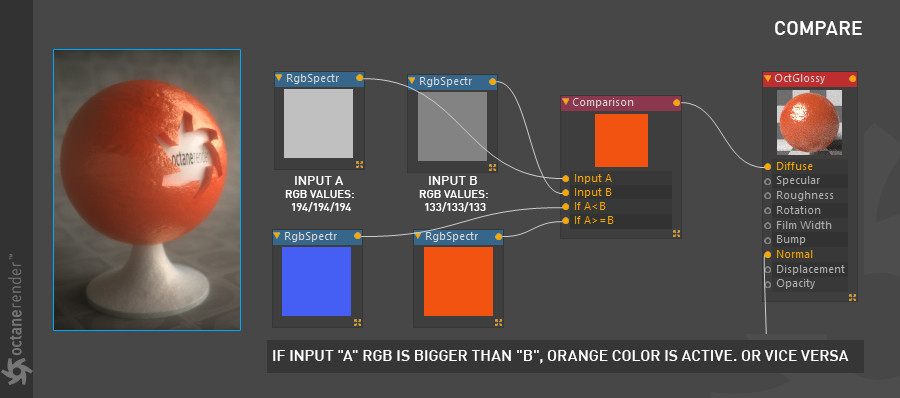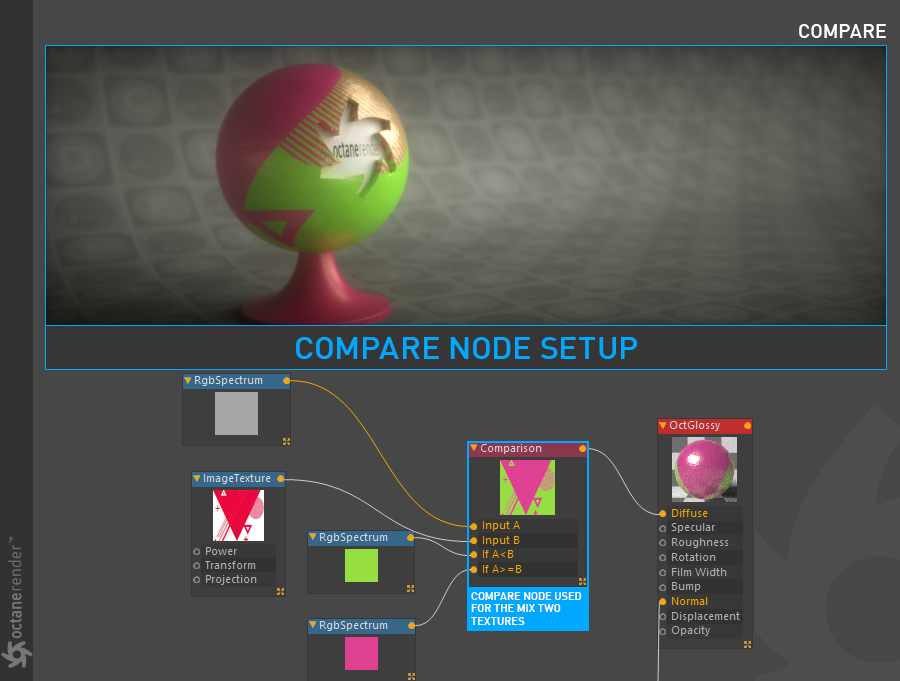# Compare

The Compare node offers you the ability to use a logical comparison operator as a way to combine textures. The node takes four inputs. The first two inputs are the textures that will be compared. The second two inputs are the result of the comparison.

The pixel values of the 2 texture to be compared are taken as reference and the result depends on the chosen logic operator (figure 1). As shown in the following example, if the input A is equal to or greater than the input B, the orange color is active. The important thing here is the numeric pixel value of the data in the Input channel. In the example, each pixel value of the input A is 194. So if we assign an RGB spectrum node to If A> = B, the result is orange. If you go through this logic and change the locations of 2 input nodes, the result will be blue.Figure 1: Two examples of using the Compare node to control the color output.